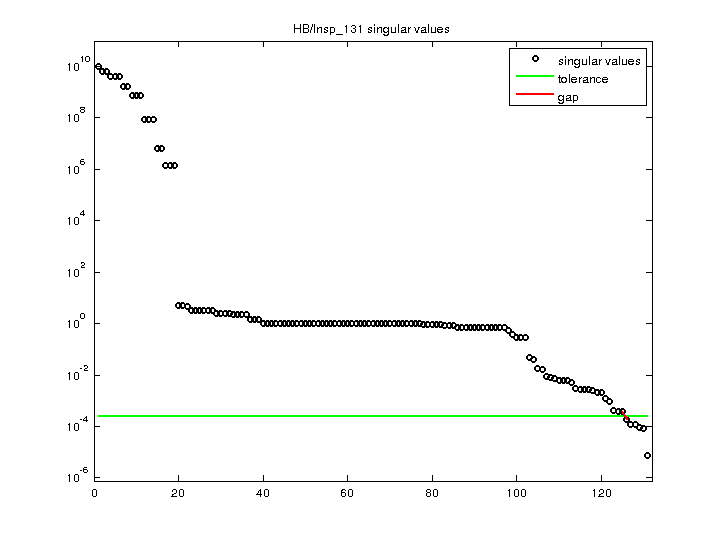Matrix: HB/lnsp_131

Description: UNSYMMETRIC MATRIX FROM I.P.J. DEC 1982. LINEARIZED N-S. COMPRESSIBLE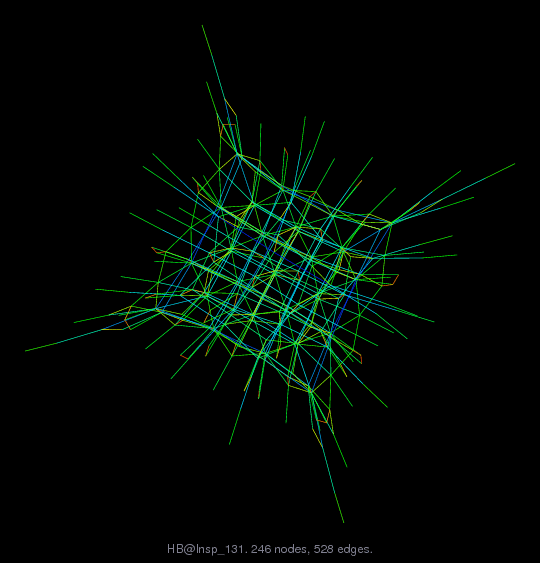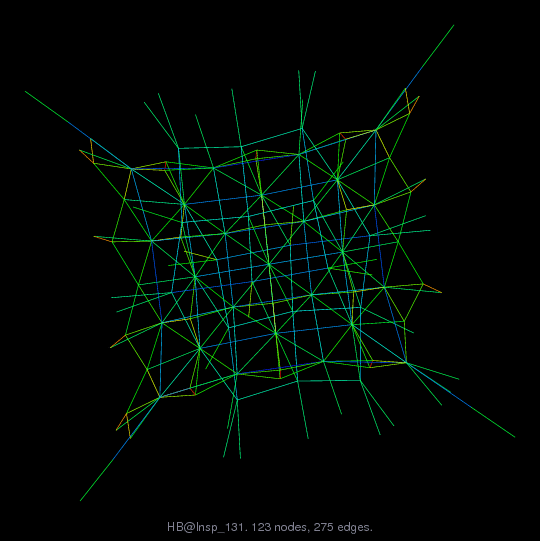(bipartite graph drawing) (graph drawing of A+A')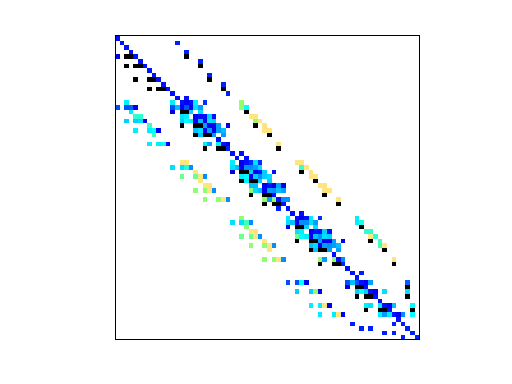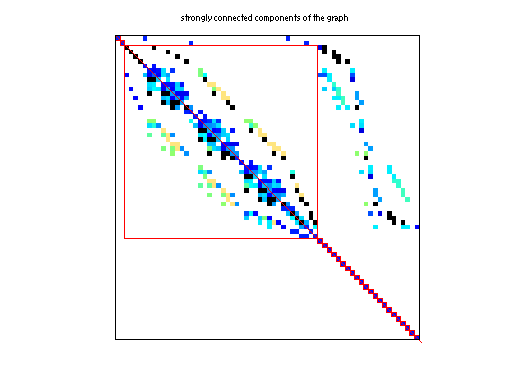• Matrix group: HB
• download as a MATLAB mat-file, file size: 3 KB. Use UFget(192) or UFget('HB/lnsp_131') in MATLAB.

 Matrix properties number of rows 131 number of columns 131 nonzeros 536 structural full rank? yes structural rank 131 # of blocks from dmperm 65 # strongly connected comp. 48 explicit zero entries 0 nonzero pattern symmetry 70% numeric value symmetry 2% type real structure unsymmetric Cholesky candidate? no positive definite? no

 author I. Jones editor I. Duff, R. Grimes, J. Lewis date 1982 kind computational fluid dynamics problem 2D/3D problem? yes

 Ordering statistics: result nnz(chol(P*(A+A'+s*I)*P')) with AMD 560 Cholesky flop count 3.4e+03 nnz(L+U), no partial pivoting, with AMD 989 nnz(V) for QR, upper bound nnz(L) for LU, with COLAMD 809 nnz(R) for QR, upper bound nnz(U) for LU, with COLAMD 1,728

 SVD-based statistics: norm(A) 9.7721e+09 min(svd(A)) 7.64219e-06 cond(A) 1.2787e+15 rank(A) 125 sprank(A)-rank(A) 6 null space dimension 6 full numerical rank? no singular value gap 1.95598

 singular values (MAT file): click here SVD method used: s = svd (full (A)) ; status: ok